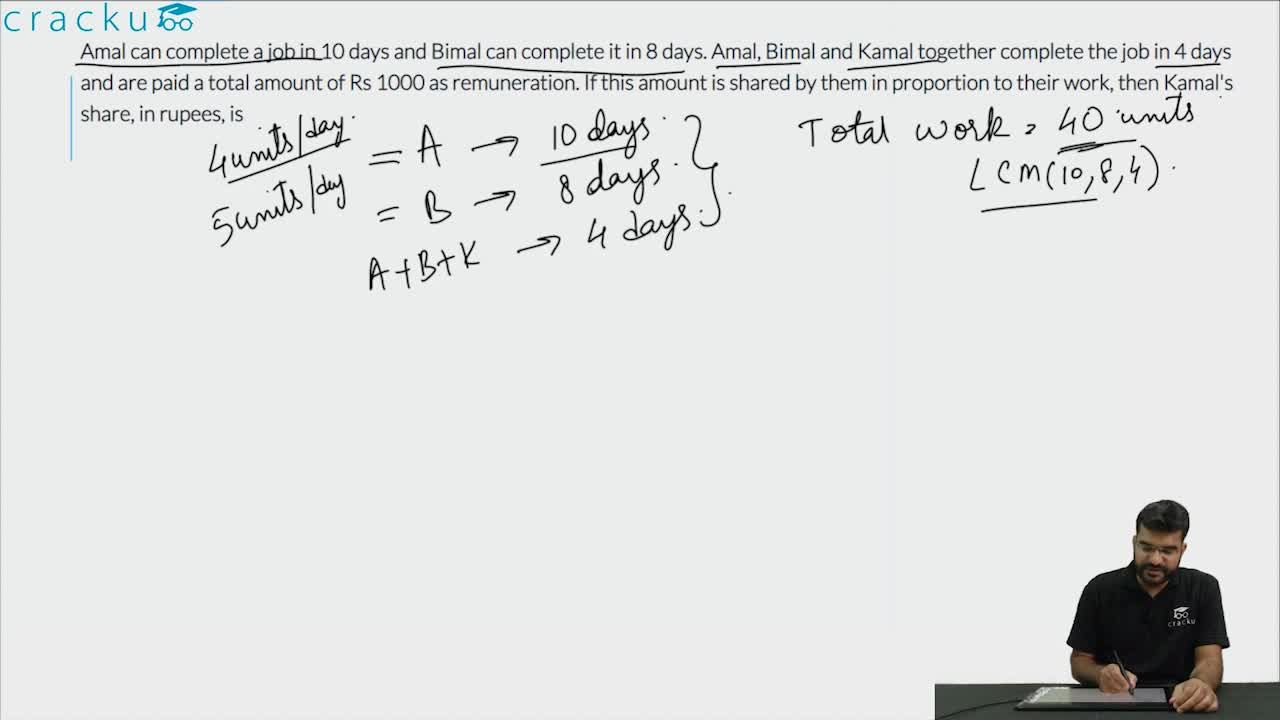Question 13

# Amal can complete a job in 10 days and Bimal can complete it in 8 days. Amal, Bimal and Kamal together complete the job in 4 days and are paid a total amount of Rs 1000 as remuneration. If this amount is shared by them in proportion to their work, then Kamal's share, in rupees, is

Solution

Let the time take by kamal to complete the task be x days.
Hence we have $$\frac{1}{10} + \frac{1}{8} + \frac{1}{x} = \frac{1}{4}$$
=> x = 40 days.
Ratio of the work done by them = $$\frac{1}{10} : \frac{1}{8} : \frac{1}{40}$$ = 4 : 5 : 1
Hence the wage earned by Kamal = 1/10 * 1000 = 100

### View Video Solution